# Geometric progression - math problems

#### Number of problems found: 128

• Railways 3Railway Corporation wants to purchase a new machine for \$360,000. Management predicts that the machine can produce sales of \$220,000 each year for the next 5 years. Expenses are expected to include direct materials, direct labor, and factory overhead (exc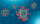A Street has 13 houses in a row. Some resident sin the first house tested positive for Covid-19. The virus spreads in 2 ways: It can spread to the next house or jump directly to the third house. Residents of house 2 can get infected in only one way, house
• GP - sequence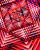The first three terms of a geometric sequence are as follows 10, 30. 90, find the next two terms of this sequence.
• Two geometric progressions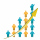Insert several numbers between numbers 6 and 384 so that they form with the given GP numbers and that the following applies: a) the sum of all numbers is 510 And for another GP to apply: b) the sum of entered numbers is -132 (These are two different geome
• Five yearsNakato Nobuki, a Japanese artist, wants to have \$24,000.00 in his savings account at the end of five years. Mr. Nobuki deposits \$1,500.00 annually into savings and has a balance of \$8,000.00 today. What must the interest rate on the savings account be for
• The blockThe block, the edges formed by three consecutive GP members, has a surface area of 112 cm2. The sum of the edges that pass through one vertex is 14 cm. Calculate the volume of this block.
• The town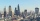The town population is 56000. It is decreasing by 2% every year. What will be the population of the town after 13 years?
• Suppose 3Suppose that a couple invested Php 50 000 in an account when their child was born, to prepare for the child's college education. If the average interest rate is 4.4% compounded annually, a, Give an exponential model for the situation b, Will the money be
• Sequences AP + GPThe three numbers that make up the arithmetic sequence have the sum of 30. If we subtract from the first 5, from the second 4 and keep the third, we get the geometric sequence. Find AP and GP members.
• Annual interest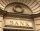A loan of 10 000 euro is to be repaid in annual payments over 10 years. Assuming a fixed 10% annual interest rate compounded annually, calculate: (a) the amount of each annual repayment (b) the total interest paid.
• Annual growthThe population has grown from 25,000 to 33,600 in 10 years. Calculate what was the average annual population growth in%?
• Annual increaseThe number of cars produced increased from 45,000 to 47,000 in 3 years. Calculate the average annual increase in cars in%.
• Find the 21Find the sum of the six terms of the finite geometric sequence 96, -48, 24, -12
• Consecutive membersThe block has a volume of 1728 cm³. Determine the lengths of the edges a, b, c of the blocks for which a < b < c and a + b + c = 38 cm and whose numerical values in cm represent three consecutive members of the geometric sequence.
• Present valueA bank loans a family \$90,000 at 4.5% annual interest rate to purchase a house. The family agrees to pay the loan off by making monthly payments over a 15 year period. How much should the monthly payment be in order to pay off the debt in 15 years?
• SavingsSuppose on your 21st birthday you begin making monthly payments of \$500 into an account that pays 8% compounded monthly. If you continue the payments untill your 51st birthday (30 years), How much money will be in your account? How much of it is interest?
• Future valueSuppose you invested \$1000 per quarter over a 15 years period. If money earns an anual rate of 6.5% compounded quarterly, how much would be available at the end of the time period? How much is the interest earn?
• Retirement annuity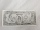How much will it cost to purchase a two-level retirement annuity that will pay \$2000 at the end of every month for the first 10 years, and \$3000 per month for the next 15 years? Assume that the payment represent a rate of return to the person receiving th
• Sum 1-6Find the sum of the geometric progression 3, 15, 75,… to six terms.
• Sum of GP members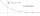Determine the sum of the GP 30, 6, 1.2, to 5 terms. What is the sum of all terms (to infinity)?

Do you have an exciting math question or word problem that you can't solve? Ask a question or post a math problem, and we can try to solve it.

We will send a solution to your e-mail address. Solved examples are also published here. Please enter the e-mail correctly and check whether you don't have a full mailbox.

Please do not submit problems from current active competitions such as Mathematical Olympiad, correspondence seminars etc...